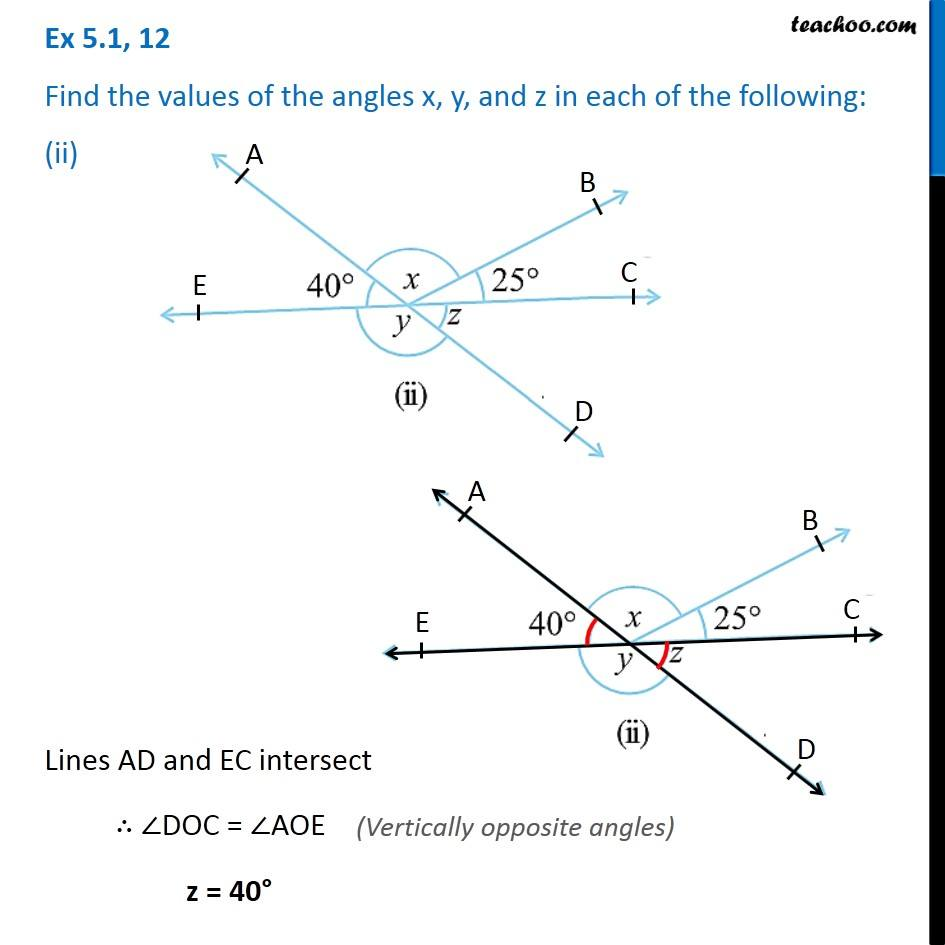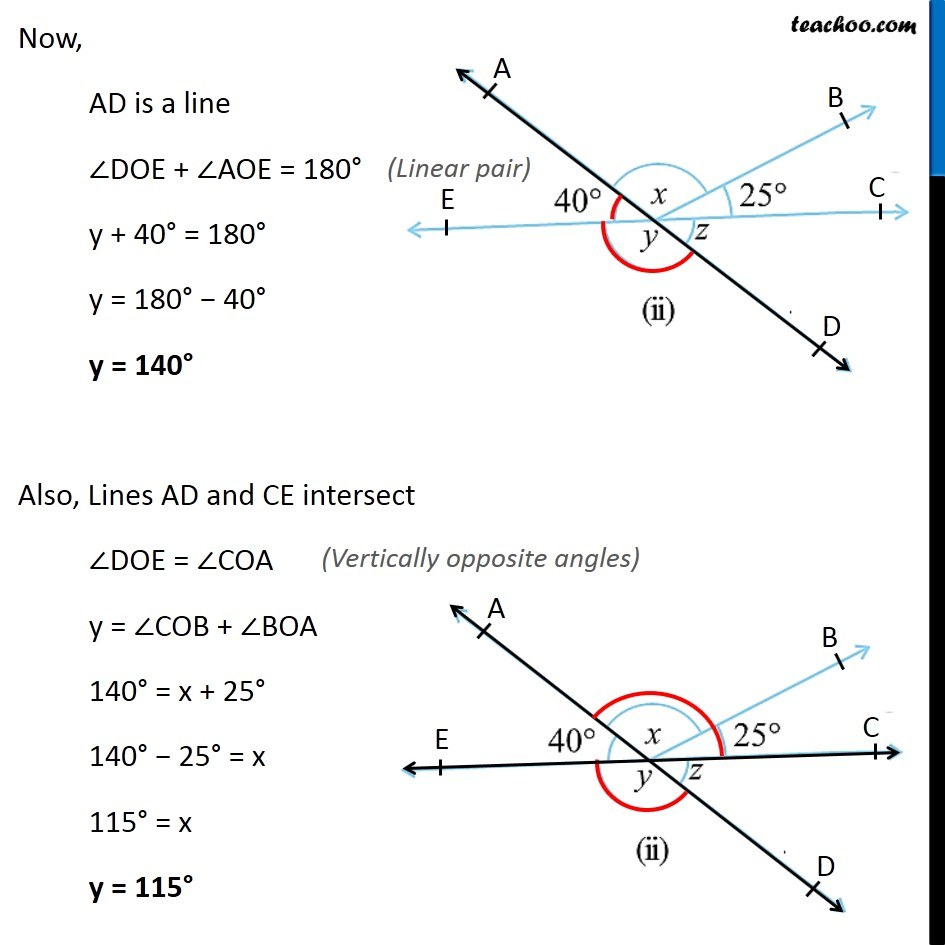1. Chapter 5 Class 7 Lines and Angles
2. Concept wise
3. Adjacent Angles, Linear Pair of angles, Vertically Opposite angles

Transcript

Ex 5.1, 12 Find the values of the angles x, y, and z in each of the following: (ii)Lines AD and EC intersect ∴ ∠DOC = ∠AOE z = 40° Now, AD is a line ∠DOE + ∠AOE = 180° y + 40° = 180° y = 180° − 40° y = 140° Also, Lines AD and CE intersect ∠DOE = ∠COA y = ∠COB + ∠BOA 140° = x + 25° 140° − 25° = x 115° = x y = 115°

Adjacent Angles, Linear Pair of angles, Vertically Opposite angles